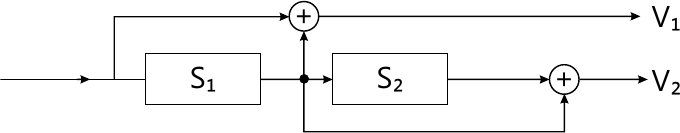MORE IN Information Theory and Coding Techniques
SPPU Electronics and Telecom Engineering (Semester 6)
Information Theory and Coding Techniques
December 2015
Total marks: --
Total time: --
INSTRUCTIONS
(1) Assume appropriate data and state your reasons
(2) Marks are given to the right of every question
(3) Draw neat diagrams wherever necessary

Solve any one question from Q1 and Q2
1 (a) Design a Huffman code for a source generating 4 different types of messages with probabilities 0.3, 0.2, 0.4, 0.1. Find the coding efficiency.
7 M
1 (b) What are Golay codes? Explain with suitable example.
7 M
1 (c) Write the procedure for coding of cyclic codes.
6 M

2 (a) A 3 bit PCM system generates 1000 samples/sec. If the quantized samples are produced by the system with probabilities ( \left { \dfrac {1}{4}, \dfrac {1}{4}, \dfrac {1}{8}, \dfrac {1}{8}, \dfrac {1}{16}, \dfrac {1}{16}, \dfrac {1}{16},dfrac {1}{16}, \right } ) Then find the rate of information. If the samples are equiprobable, what will be rate of information?
7 M
2 (b) What are Hamming codes? Explain with suitable example.
7 M
2 (c) For a (7,4) cyclic code, with generator polynomial g(x)=x2+x2+1, what will be code what for following message words.
i) 1011
ii) 1110.
6 M

Solve any one question from Q3 and Q4
3 (a) Find the generator polynomial for BCH code with codeword length n = 15 and error correcting capability tc=2.
10 M
3 (b) Explain Go-Back-N ARQ.
6 M

4 (a) For a (7,5) RS code, the received codeword polynomid is given as: r(x)=x55x42x3+x26x+a3 where α is element of aF(23). Find the corrected codeword polynomial, if there is single error in the received codeword.
8 M
4 (b) Write features of BCH codes.
4 M
4 (c) What is FEC & ARQ systems?
4 M

Solve any one question from Q5 and Q6
5 (a) Draw the trellis diagram for following encoder.8 M
5 (b) Explain with example polynomial description of convolutional codes.
8 M
5 (c) Draw the block diagram for coding process Turbo codes.
2 M

6 (a) Explain Viterbi's algorithm for decoding of convolutional codes.
8 M
6 (b) Explain generating function for convolutional codes.
8 M
6 (c) Write any two features of LDPC codes.
2 M

Solve any one question from Q7 and Q8
7 (a) What are the goals of communication system designer? Explain any three of them.
6 M
7 (b) What is Nyquist minimum bandwidth?
4 M
7 (c) Explain in brief trade off between modulation and coding.
4 M

8 (a) Explain how coding gain is improved in TCM.
8 M
8 (b) Write shannon-Hartley capacity theorem. What are its implications?
8 M

More question papers from Information Theory and Coding Techniques